Reach Us+32-10-28-02-25
The Quantum Sieve of Eratosthenes | OMICS International
Journal of Physical Mathematics
All submissions of the EM system will be redirected to Online Manuscript Submission System. Authors are requested to submit articles directly to Online Manuscript Submission System of respective journal.

The Quantum Sieve of Eratosthenes

Sowa A*

Department of Mathematics and Statistics, University of Saskatchewan, Canada.

*Corresponding Author:
Sowa A
Professor
Department of Mathematics and Statistics
Tel: 154445354154
E-mail: [email protected]

Received Date: April 08, 2016; Accepted Date: June 22, 2016; Published Date: June 27, 2016

Citation: Sowa A (2016) The Quantum Sieve of Eratosthenes. J Phys Math 7:180. doi:10.4172/2090-0902.1000180

Copyright: © 2016 Sowa A. This is an open-access article distributed under the terms of the Creative Commons Attribution License, which permits unrestricted use, distribution, and reproduction in any medium, provided the original author and source are credited.

Visit for more related articles at Journal of Physical Mathematics

Abstract

We introduce and examine quantum states of a special kind, referred to as E-states, whose properties are both structurally and functionally analogous to the sieve of Eratosthenes. More broadly, the concept of an E-state is related to a certain noncommutative extension of the Dirichlet ring, also discussed here for the first time. Furthermore, we demonstrate that E-states can be implemented on a universal quantum computer and, as a particular application, we construct an algorithm which implements the Dirichlet multiplication of sequences on a quantum computer. We also discuss the potential applicability of E-states to the problem of integer factorization although, we haste to add, we are not aware at present of the possibility of using this approach to obtain algorithms of sub-exponential complexity

Keywords

Quantum sieve; Quantum algorithm for Dirichlet multiplication (convolution); Integer factorization

Introduction

Quantum parallelism is a phenomenon that Nature herself seems to be suggesting as a means to getting around repetitive chores of forbidding duration. It is the cornerstone of most if not all interesting quantum computing schemas and the main factor responsible for computational speedups of some quantum algorithms, e.g. Shor’s pioneering integer factorization algorithm, . Arguably, quantum parallelism may be so distinct a feature of quantum algorithms that the efficiency some of them provide will remain unachievable within the Church-Turing’ian model of computation [2,3].

In this article we explore possible advantages of some quantum structures deemed analogous to the classical sieve of Eratosthenes. The main idea is to set a bipartite quantum system in a state of the form Σalk|l⟩ k⟩ with amplitudes alk nonzero only if k|l (i.e. l can be divided by k without remainder). We refer to such states as Eratosthenian states or, simply, E-states. If we were skilled in the art of creating E-states with good control they could be used for efficient integer factorization. Suppose we wanted to find a divisor K of N, where N is a number with n~log N bits (in binary representation). All we would have to do is implement an E-stare with only a polynomial in n quantity of comparable-magnitude amplitudes alk≠0, including aNk ≠0. If such a state were created we would be able to find the factor K of N with only a polynomial in n number of trials. Note that for a construction of such a state K need not be known a priori. Instead, it would suffice to ensure that the state is an E-state and that its nonzero amplitudes would have the first index concentrated near N, which is much more generalist a description. The challenge is to find ways of implementing such states. In Section 3 we propose several ways of implementing E-states on a quantum computer. Unfortunately all the methods we have found thus far result in a number of nonzero amplitudes that is exponential in n. However, it is not clear if this should be inevitable. Is there a fundamental constraint preventing successful realization of E-states with amplitudes concentrated in small areas of interest? For now this is an open question. A sceptic might argue that perhaps the whole idea should be dismissed as backward, given that a superior algorithm is already known—namely, the algorithm of Shor. However, we take an opposing view. Indeed, we find the concept to be intriguing for at least these three reasons: First, we do not exclude the possibility that future research could bring a discovery of a method enabling construction of very rarefied E-states with only a polynomial in n number of nonzero amplitudes placed in targeted places. Alternatively, in time we may be able to understand the fundamental reasons why this is impossible or intractable, e.g. by finding arguments for uncomputability of such states or at least a revealing constraint on the required physical resources. Second, as demonstrated in subsection 3.4, the concept of E-states enables implementation of the Dirichlet multiplication on a quantum computer. This new algorithm is interesting in its own right. Third, as shown in Subsection 2.2 the set of E-matrices (a notion slightly more general than that of E-states, the latter necessarily requiring normalization) forms a noncommutative ring, which extends the classical Dirichlet ring. We feel that an occurrence of a fundamental and, to our knowledge, entirely new algebraic structure further strengthens the case for E-states.

It is one of the conclusions reached in this paper that the algebra of the Dirichlet polynomials — i.e. objects of the type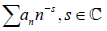, where the sum is finite — may be handled by a quantum computer. This extends our earlier work in which we demonstrated that the Dirichlet polynomials may be efficiently manipulated on a classical computer. In both cases the manipulation of Dirichlet polynomials is enabled by their matrix representation (which also extends to the infinite Dirichlet series). This fact has numerous practical applications [4-6].

Eratosthenian Matrices and Eratosthenian Quantum States

E-matrices

Consider a matrix A=[ank], and either n,k∈{1,2…N} or both indices run over the entire set of positive integers N. We will say that A is Eratosthenian (an E-matrix for short) if and only if.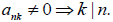When written explicitly an E-matrix assumes the form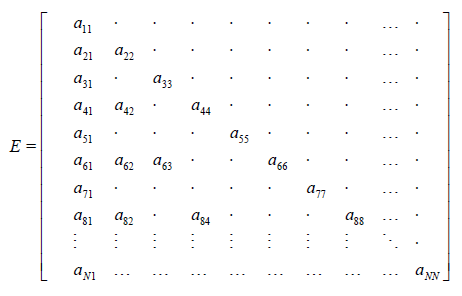where the entries represented by centred dots are necessarily zeros, and only if k|n the entry ank may be nonzero. If an E-matrix E is N-by N, we write E∈εN and if it is infinite, extending indefinitely to the right and down, we write E∈ε. Note that the number of nonzero elements in the n’th row of an E -matrix is at most d(n) (i.e. the number of divisors of n). For E∈εN a the overall number of nonzero entries is at most σ (N) = d(1) + d(2) …+ d(N) = O(N log N) , .

It is demonstrated in Subsection 2.2 that every εNN∈{2,3,4…}∪{∞}, is a ring with respect to the regular matrix addition and multiplication. We also point out that every εN contains a nontrivial commutative subring. To see this we introduce the following definition. Namely, we say that an E-matrix is a Dirichletian matrix (D-matrix for short) if ankn/k for a sequence[α1, α2…] which may be finite or infinite. We let DN, N∈{2,3,4…}∪{∞} denote the set of all N-by N D-matrices. It is easily seen that DN is a commutative subring of εN. Also, it is known, see , that is isomorphic to the Dirichlet ring, i.e. the ring of infinite sequences with component-wise addition and the Dirichlet multiplication1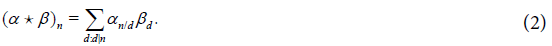Therefore, ε furnishes a noncommutative extension of the Dirichlet ring.

E-matrices form a noncommutative ring

Readers who are interested only in the quantum-computing applications of E-matrices may skip this subsection.

Theorem 2.1: Let N∈{2,3,4…}∪{∞}.N is a ring with respect to the operations of matrix addition and multiplication. The ring has a unity given by the identity matrix I. For A,B∈εN, AB=I if and only if BA=I. Moreover,A∈εN has an inverse (in εN) if and only if all the diagonal entries of A are nonzero.

Proof: It is ion. Next, we verify that the product of two E matrices is an E-matrix. Indeed, for A,B∈εN, let[cnk]=C=obvious that εN is a group with respect to matrix addition. Furthermore, the identity matrix clearly is the unit of multiplicatAB. We have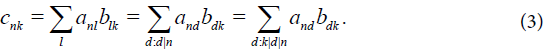(Here,k|d|n is used as shorthand for: k|d & d|n.) Thus, for the righthand side sum to be nonzero at least one of the terms must be nonzero, which implies k|n, i.e. C is an E-matrix.

It follows from (3) that if A,BεN and AB=I, then annbnn=1. Thus, for a matrix A∈εN to have a left or right inverse in εN it is necessary that all its diagonal entries be nonzero. Next, let A=[ank]∈εN and ann≠0 for all n. We will construct B∈εN such that AB=I. Indeed, suppose: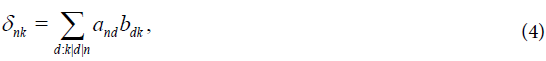Where δnk∈{0,1} is the Kronecker delta. This determines the diagonal entries of B, namely, bnn=1/ann for all n. In addition, if k|n, k<n, then (4) implies:Note that (5) determines bnk via bmk (k|m) with m<n. This furnishes a recurrence formula for the off-diagonal entries of B that is the right inverse of A. If A is finite it is clear that B is also the left inverse, i.e. BA=I. Therefore, in the rest of the proof we assume that A is infinite. We will find its left inverse C∈εN, i.e. CA=I. As before, assume: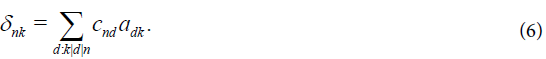It follows that Cnn=1/ann for all n. Furthermore, if k<n, k|n, (6) implies: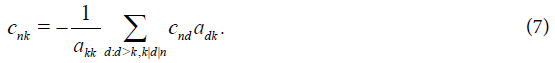This gives recurrence for cnk if the indices are suitably ordered. Namely, if l|m and k|n we say (m,l)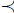(n,k). if either m<n or m=n and l>k. Observe that in (7) cnk is determined via cnl with (n,l)(n,k). Thus construction by recurrence yields C such that CA=I. Finally, note that C=CI=CAB=IB=B , i.e. the left and the right inverses of A are equal. This completes the proof.

Corollary 2.1: The ring, εNN∈{2,3,4…}∪{∞}, is not local.

Proof: In light of Theorem 2.1 we see that the set of non-invertible elements in εN (N>2) or ε is not additively closed. Indeed, the sum of two E matrices each with some zeros on the diagonal can yield a matrix whose all diagonal entries are all nonzero. The claim then follows from Theorem 7.1.1 in .

Remark 1: Note that the set of all matrices in εN (or ε) with a zero column (resp. zero row) is a left (resp. right) ideal. The fact that a ring is not local means that the set of non-invertible elements is not a twosided ideal. Moreover, neither of the rings has a largest proper left or right ideal, .

Remark 2: Note that the recurrence formulas (5) or (7) yield the inverse matrix by filling up consecutively increasing blocks. This can be viewed alternatively in the following way. A matrix A∈εN has a block structure as follows: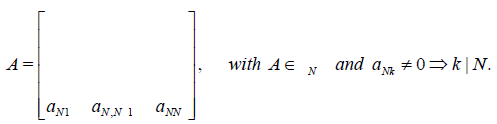Suppose det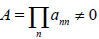, and let us look for A-1 in the form,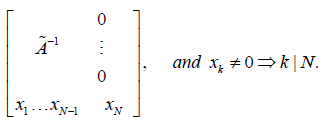In such a case A-1A=I is equivalent to the following set of constraints: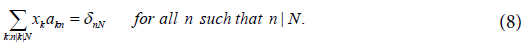Let X=[x1,…xd,….xN] be the vector whose entries are xd with d|N in the natural order. Observe that (8) has the form XM= [0,…1] where M is a suitable upper triangular d(N)-by-d(N) matrix, and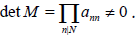Therefore X=[0,…1]M-1 is the unique solution of (8).

Quantum E-states

Consider a pair of quantum systems which, when in isolation, have the dynamics determined by the Hamiltonians

Hi :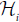(i=A,B), whererepresent the respective Hilbertspaces of states. Let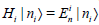denote the eigenbasis induced by Hi, i.e.. For our purposes it is necessary to assume that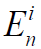, so that all the eigenspaces of Hi are 1-dimensional. When the mutual isolation of the quantum systems at hand is violated, the pair need to be considered as a composite (more specifically, bi-partite) system. Now, the individual states no longer hold any meaning and are superseded by a composite state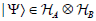whose dynamics, in the simplest possible case2, is determined by the composite system Hamiltonian,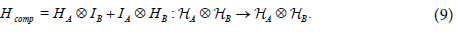Suppose the composite system is initialized in the state,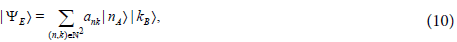Where E=[ank] is an E-matrix with the Hilbert-Schmidt norm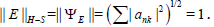The Schrödinger dynamics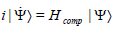with the initial condition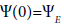amounts to a separable evolution of the coefficients and so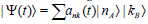, where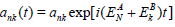. This means that if |Ψ(t)⟩ is represented in the |nA⟩|kB⟩ basis via an E-matrix at one time, it will remain to be represented by an E-matrix for all times. It is therefore justifiable to call an evolving state as this an E-state. Since time evolution of the phase factors is inconsequential for the discussion that follows we will make no further reference to it.

Quantum measurements and integer factorization

Suppose an E-state |Ψ(E)⟩ has been prepared in such a way that ank≠0 ⇒ k|n. Suppose we wish to factor a specific integer N. A measurement of the composite system energy returns a pair ((n,k)) where with probability one k|n. However, the probability of drawing such a pair is exactly |ank|2. This means that if all |ank| have comparable magnitude, we need at least σ(N)=O(NlogN) trials to find a specific pair — the number of trials is exponential in log N or exponential in the number of bits used to represent N. This would change if we were able to prepare the system in an E-state concentrated on the components of the form |nA⟩|kB⟩ where k|n. This is, of course, a hard problem. We discuss it from the point of view of quantum computing in Section 3. ((n,k))

E-states on a quantum computer

In this section we consider two different approaches to the construction of an E-state on a quantum computer. The first method relies upon the quantum multiplication circuit, while the second utilizes a quantum gate implementation of a specific arithmetic function. In both cases the resulting state is a superposition of a pure E-state with some additional “artefact" state components. However, as explained below, the pure E-state components are separated from the artefact components by their index range. Thus pure components can be separated a posteriori by a rudimentary classical observation or, alternatively, enhanced via the procedure known as amplitude amplification, .

The departure point for both constructions is the known process for creating an equal superposition state, see e.g. . This is obtained by an application of the single qubit Hadamard gates to the ground state: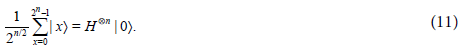An E-state from quantum multiplication

We will demonstrate how to prepare an E-state on a quantum computer with a quantum random access memory consisting of two registers. We let |x⟩ |y denote the state of the registers, where it is understood that |x⟩=|x1,x2,x3,..xn⟩ is an n-qubit representation and, similarly, |y⟩=|y1,y2,y3,..yn⟩. Well known are quantum computing implementations of certain basic arithmetical operations, see e.g. [11,12] including addition and multiplication. We will make use of the multiplication circuit, implementing |x⟩=|x1,x2,x3,..xn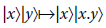Where x.y denotes the product of two integers. (Here, multiplication may be effectively replaced by multiplication mod 22n.) However, since multiplication by zeros is not interesting we introduce a modification. Namely, we first add 1 to both x and y, effectively using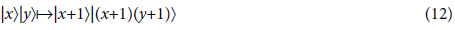Note that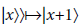is unitary, and implementable as simplified addition, as long as the register is by one qubit larger that the maximal value of x. Similarly, unitarity implies that |x+1⟩|(x+1)(y+1)⟩ cannot fall off the register range. Therefore, the second register should have at least M=22n qubits. We will now combine (11) with (12) to create an E-state. Indeed, in step one we set both registers in the equal superposition states: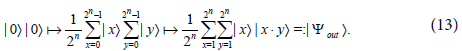The output state is very similar to an E-state except for some artefacts. The artefacts can be effectively dealt with either by treating them as a tolerable nuisance, and doing nothing until the quantum information is accessed, or else countermanding them via an application of the Grover’s search (amplitude amplification). We illustrate the procedure by an example with n=2, and M=n2=4. Note that the first register must support n+1=3 qubits. The end state in this case has the form (here c=1/4):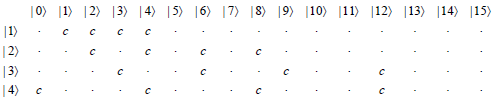Note that not shown are rows corresponding to the states |5⟩,|6⟩,|7⟩ or |0⟩ on the first register—these rows contain only zero entries. Furthermore, note that the submatrix corresponding to values |1⟩,|2⟩,|3⟩,|4⟩ on the second register is the transpose of an E-matrix3. We also note that a measurement on both registers returns a pair of integers (a,b) where, a|b. (In the example at hand the special pair (4,0) signifies (4,16) .Note that those components of the output state that correspond to values other than |1⟩,|2⟩,|3,|4⟩ on the second register cannot be erased, because such an operation would not be unitary. However, the resulting state contains an E-state as a separable component. Summarizing, the proposed algorithm for the creation of an approximate E-state consists of the following steps:

• Prepare an initial state according to (11).

• Compute the (shifted) quantum product according to (13). As a result obtain an output state |Ψout⟩=|ΨE⟩+|ΨR⟩, where |ΨE⟩ is (proportional to) an E-state and |ΨR⟩ is a remainder.

• Separate |ΨE⟩ classically in the following sense: the measurement outcomes that belong in an E-state component are precisely those |x⟩|z⟩ where z∈{1,2…2n}

• Optionally, depending on an application, it may be beneficial to engage the generalized Grover’s search as a processing step preceding the classical separation. This step provides amplitude amplification, effectively boosting the weight of the |ΨE⟩ component and suppressing that of the |ΨE⟩.

Remark 1: Note that the probability of an outcome that is an E-state component is (d(1) + d(2) +d(2n )) / 22n~2n log 2n /22n~n / 2n . However, measurements on the full output state provide useful information, and separation of the E-state pointed out in the last step of the algorithm need not always be desirable. We emphasize that the probability of obtaining from measurement any pair a|b is always the same and equal to 2-2n.

Remark 2: The network complexity for multiplication of two registers is of the order n2. There are several known circuit architectures implementing multiplication. While some implementations rely on the quantum Fourier transform, , the classical Vedral-Barenko- Ekert algorithm does not use it, . Even though the latter algorithm demands a greater memory resource, it seems to secure computational stability independent of the input size.

Remark 3: Recall that the Grover search, as well as its various generalizations known as the amplitude amplification algorithms, , rely on the Grover rotation. To briefly summarize the principle and compute the complexity, we turn attention to |Ψout⟩=|ΨE⟩+|ΨR⟩. The quantities a=⟨EE⟩ and b=⟨ΨRR⟩ represent the probabilities of measuring a good component, in the support of |ΨE⟩, and a bad component in the support of |ΨR. We wish to apply a quantum circuit to |Ψout⟩ so as to boost the probability of measuring the good component. To this end we use the Grover’s rotation: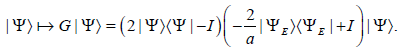Grover’s rotation is applied k times to |Ψout⟩ so that Gkout⟩ approaches a-1/2E⟩. The value of k depends on the initial partition of energy between |ΨE⟩ and |ΨR⟩ or, in other words, the ratio of the number of good states to the number of bad states. We have,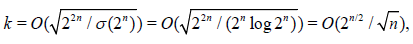i.e. the number of iterations is essentially exponential in n.

An E-state from f(x)=[N/x]. x.

This method is based on a quantum implementation of the arithmetic function f(x)=[N/x]. x, x∈N. (We will not discuss the specific circuit implementation of this function, all reversible classical functions can be converted into quantum computations . We wish to construct an E-state concentrated near the N’th column, where N<2n. To this end we prepare the state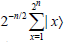on the first register and subsequently implement a two-register operation.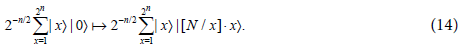The result is a relatively sparse E-state in superposition with an artefact vector. Let us illustrate this with an example for N=6. Selecting n=3, we obtain the following state matrix ( c =1/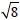):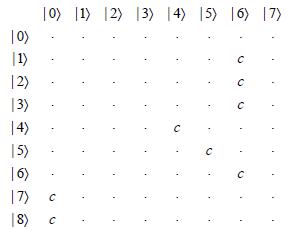Note that the first register requires 4 qubits, and only part of it is shown. The E-state submatrix is indexed by |1⟩……|6 on both registers. As before there is some overflow of energy to artefact state components, in this case, c|7⟩|0⟩+c|8⟩|0⟩. As before the artefact components can be separated classically a posteriori or suppressed via amplitude amplification.

Note that in general a measurement will result in drawing a nontrivial divisor of N with probability 2-n(d(N)-2). Again, one may apply the Grover’s rotation in order to bring the state close to the state supported on the d(N)-2 good components, which requires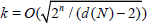iterations.

Applying the quantum Fourier transform

Let us briefly examine the effect of an application of the quantum Fourier transform (QFT) to an E-state. In the next subsection we will discuss the topic from a more general point of view, but it is helpful to first focus on the E-state part of |Ψout⟩=|ΨE⟩+|ΨR⟩ as in (13). As remarked above, |E⟩ is separated from the remainder |ΨR⟩ by the second register range 1≤z≤2n. We will refer to the |ΨR⟩ term as ‘out of bounds’ (OOB). Thus,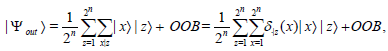Where δ.|z(x)=1 if x |z and δ.|z(x)=0 otherwise. Recall, , that the n-qubit QFT is defined via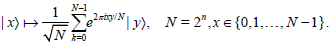Applying the shift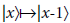on the first register, and setting fz(x)=δ.|z(x+1) followed by the QFT yield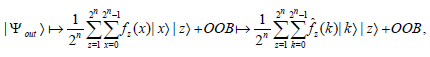where for all z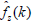is the discrete Fourier transform of fz. It is interesting to observe that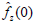= 2-n/2d(z). Therefore, if the output state has been prepared as this, then from among the output states of the form |0⟩|z⟩, the more highly composite numbers, i.e. those with larger d(z), are more likely to be measured. [β1, β2..]T =A[α1, α2,…]T

The Dirichlet product of state vectors

In recent years there has been a trend in quantum computing to go beyond the realm of discrete algorithms and consider the possibility of manipulating the vectors represented in quantum state amplitudes. A representative example of that trend is reference , wherein the authors address the problem of solving on a quantum computer the linear equation [β1, β2..]T =A[α1, α2,…]T. Here |α⟩=Σαx|x⟩ is regarded as given, A is a classically known matrix, and

|β⟩=Σ βx|x⟩ is to be computed. Of course, prerequisite to this procedure is the possibility of implementing on a register the state |α⟩ with the particular set of amplitudes. One method for accomplishing that is indicated in . We base the following discussion on the possibility of implementing a state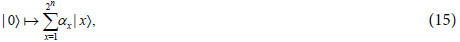Where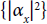is a probability distribution4 on {1,2,…2n} With this understood, we will now show how to use the concepts introduced in the preceding paragraphs to describe an implementation of new types of E-states as well as the realization of the Dirichlet multiplication of the amplitude vectors. First, consider a straightforward generalization of (13). Namely,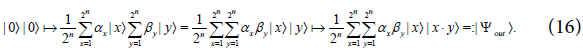Let us examine an example, assuming n=2, and M=n2=4 (M=4 is the number of qubits required for the second register, and n+1=3 is the number of qubits required for the first register.) In such a case |Ψout⟩ assumes the form: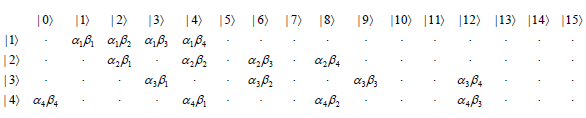Note that the 4-by-4 submatrix delineated by the vertical lines supports an E-state component. In general, we have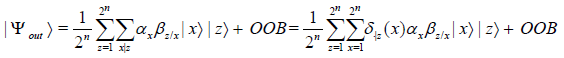This is much more general an E-state than that obtained in (13).

Next, we perform further computation of the output state. We first shift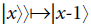on the first register, and then follow by the QFT on the first register. In detail, by letting fz.|z(x+1)αx+1βz/(x+1) we obtain,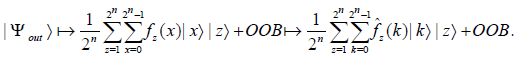Finally, we observe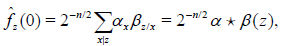Where α⋆β is the Dirichlet product (convolution) of the sequences α and β Thus, the component of |out⟩ distinguished by |0⟩ on the first register is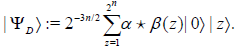Note that the example considered in subsection 3.3 is a special case and returns d(z)=ee(z), where e=[1,1,1,..]

Summarizing, the amplitudes at Gkout⟩ at |0|z⟩ components hold information about α⋆β(z). This type of information can only be accessed statistically. We point out yet again that the amplitude amplification via the Grover’s search algorithm may be engaged to obtain Gkout⟩~|ΨD⟩. The number of iterations is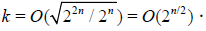Remark: We recall that the known QFT algorithm has Θ(n2) complexity. Of course, since the QFT depends on unitary transforms with terms like exp 2π /2n its hardware implementation requires precision (in terns of energy control or ultra-short pulse control, etc.) that is exponential in n. Notably, however, in some applications this difficulty may be overcome by replacing the QFT with the approximate quantum Fourier transform (AQFT) introduced in . It is interesting to enquire whether the AQFT might facilitate additional gains also in the task of integer factorization via E-states. However, we do not undertake to address this problem here.

Remarks on E-states of quantum systems in thermal bath

As explained in Section 2.3 once an E-state of the form (10) is formed, it will be preserved essentially unchanged in time (except for the unessential phase factors) as long as no measurement is conducted on the composite system. In this section we consider the problem of forming such a state outside the framework of the universal quantum computer. Indeed, one might hope that abandoning the constraint of universality would open more options for achieving such a goal. The approach we consider is based on the thermodynamic equilibrium, and utilizes the results for another application of those results. [17,18]

For simplicity we henceforth assume that |ΨE⟩ is effectively finite. The first observation is that an E-state may be reduced to a more standard state via the Schmidt representation. Indeed, let E=USV be the SVD decomposition of the matrix E=[ank] that holds the amplitudes of |ΨE⟩. Thus, U and V are unitary matrices while S=diag[s1,s2,s3,..] is a diagonal matrix. Let us define new orthonormal bases for the two subsystems, namely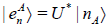, and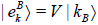. This gives the Schmidt representation of the E-state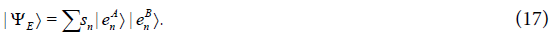We will use these observations to attempt a construction of a quantum system regime in which |ΨE⟩ will become its stationary state. The basic concept of adiabatic computing is to switch from the regime that instils |ΨE⟩ back to the dynamics governed by the Hamiltonian (9) not disturbing the system state. To this end, one might try to construct an isolated system Hamiltonian for which (17) is a ground state. However, we propose an alternative approach, based on thermodynamic equilibrium at constant temperature. First, we define a Hamiltonian5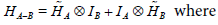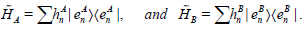Since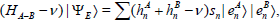E⟩is not a stationary state with respect to HA-B, unless the Hamiltonian is completely degenerate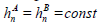. Unfortunately, the completely degenerate Hamiltonian is useless in distinguishing a specific state, since it views any state as stationary. However, consider as an alternative the evolution of the system stabilized by a heat bath at constant temperature T. When the system is in equilibrium, the Helmhotz free energy will be minimized. The Helmholtz free energy is given by: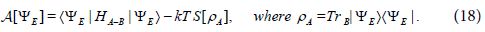Here, SA]= -TrAlogρA] is the von Neumann entropy. Note that SA]= SB] because the entropy depends on the nonzero eigenvalues of the mixed state which are equal for both subsystems. Also, since TrA]=1=const, we may assume without any change to the dynamics that S(x)= -x log x+ x ,so that -S’(x)= log x. Now, ΨE will be stationary with respect to the functional A provided it satisfies the Euler-Lagrange equation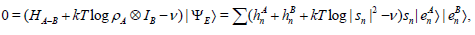and this implies: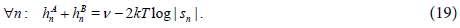Recall that {sn} is constrained by the fact that ΨE is an E-state in the basis |nA⟩|kB⟩, perhaps an E-state with specific features. This imposes a constraint on the energy levels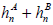. Conversely, if the bipartite quantum system is exposed to a constant temperature T regime with the internal energy HA-B, where HA-B is characterised by the precise values ofobtained via (19), the system will settle in a stationary state of A. Such a state is then expected to be an E-state w.r.t. the |nA⟩|kB⟩ basis. However, the following questions appear to have fundamental significance as regards feasibility of creating an E-state via the proposed process: First, could the SVD data of an Eratosthenian state be known a priori and explicitly? Second, even with the assumption that the SVD data are known a priori, would it be possible to build a machine that instils a regime described by (18) with the precise values ofobtained via (19)?

Discussion and Results

We have discussed novel algebraic and computational structures based on the concept of Eratosthenian matrices (E-matrices) and Eratosthenian quantum states (E-states). We have observed that E-matrices furnish a noncommutative extension of the classical Dirichlet ring. We have also considered the task of initializing and manipulating E-states on a quantum computer and introduced an algorithm that implements the Dirichlet product of quantum state vectors. In addition, we have pointed out that if a method was known for efficient initialization of E -matrices with targeted narrow supports it would facilitate efficient factorization of integers.

Acknowledgements

I acknowledge the support of the Canadian Foundation for Innovation, grant LOF # 22117. I am grateful to an anonymous reviewer of this work for the suggestion of the relevance of the approximate quantum Fourier transform.

1Note that DN with N finite inherit the Dirichlet multiplication with obvious modifications. These are the only objects that one can hope to implement numerically.

2An example of this type of a quantum system is a decoupled spin-pair in an NMR experiment.

3The transposition stems from the fact that we adhere to the conventional organization of the quantum circuit operations. A reversal of the order of the registers results in an E-matrix as defined in Section 2.

4In fact a harder problem is considered in , which is implementation of a state where |αx|2 stems from a discretization of a continuous probability distribution. Also, the reader will note that we have modified the original design by the usual shift of the register index.

5We emphasize that HA-B is to be distinguished from Hcomp.

References

Select your language of interest to view the total content in your interested language

Article Usage

• Total views: 9520
• [From(publication date):
June-2016 - Oct 22, 2019]
• Breakdown by view type
• HTML page views : 9296Can't read the image? click here to refresh